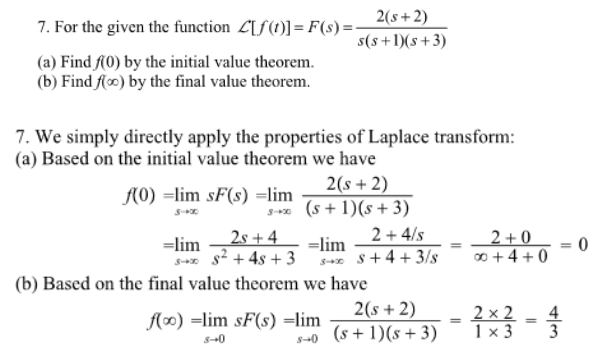For the given function, F(s)=2(s+2)/(s(s+1)(s+3)), (a) find f(0) by the initial value theorem, (b) find f(infinity) by the final value theorem.For the given function, F(s)=2(s+2)/(s(s+1)(s+3)), (a) find f(0) by the initial value theorem, (b) find f(infinity) by the final value theorem.

System Dynamics Page 2 dynamics dynamics dynamics dynamics dynamics dynamics dynamics System dynamics Page 3